• 在一个矩形方格中，随机地涂表格，求所涂多边形周长。  我们如果去求图中所有黑点所组成的多边形周长呢？小学我们就学过切割法。对于凸边形来说，其周长可以等价于一个大矩形的周长。如下图所示 ====》 即...
问题提出：
在一个矩形方格中，随机地涂表格，求所涂多边形的周长。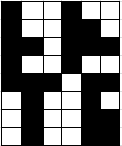我们如果去求图中所有黑点所组成的多边形的周长呢？小学我们就学过切割法。对于凸边形来说，其周长可以等价于一个大矩形的周长。如下图所示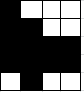====》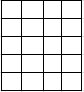即周长为4*5=20
但对于凹多边形来说，这就不对了，对于这个凹字就可以看出2*(4+5)！=18。那么应该怎么求呢？对于普通程序员来说，最熟悉的是遍历了，但我可以说的是，有更简单的方法。
我们来分析下，每个小方块矩形有4条边，但相邻的方块会有重复的边。如果我们把重复的边减掉，不就可以得到其周长了吗。先举个例子。对于两个小方块来说，重合的边为2，故其周长为2*4-2=2*(1+2)=6。其实认真想想也很容易想明白的。好了，那我们怎么求重合的边数呢？如果我们把每个小方块黑点当成一个个的点，然后相邻的黑点连成线，那就是我们所说的图了。所重合的边数，就是这个图边数的两倍，即2e(e为此图边的个数)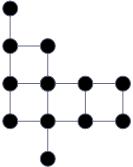根据图论的知识，所有点的度之和为边数的2倍，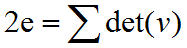。设黑点数为n，则周长为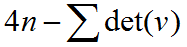。我们来上图中的周长：4*12-（1+3+2+3+4+3+2+2+4+3+2+1）=48-30=18。
以上方法所计算的结果是包含内点的，如第一幅图中右下角有一个内点，即白点周边全是黑的。如果不想包括内点，那么可以先进行对内点处理，全变成黑点，然后再处理。对于第一幅图，如果计算内点的话，即：4*25-（1+2+3+2+2+1+3+2+2+1+1+1+3+3+1+2+3+2+2+3+2+1+1+2+2）=100-48=52。而根据切割法原理，我们可以得到周长为2*（2+4）+2（3+4）-2+2（3+4）+2（4+2）+2=12+14+14+12=52。
代码为：

int perimeter(int **d,int n)
{
//	Print(d,n);
int director={{1,0},{0,-1},{-1,0},{0,1}};//方位，右-上-左-下
///预处理/
//将内点赋1
bool isOne=true;
for(int i=1;i<n-1;i++){
for(int j=1;j<n-1;j++){
if(d[i][j]==0){
isOne=true;
for(int k=0;k<4;k++){
if(d[i+director[k]][j+director[k]]!=1){//如果有一个方位不为1，则此点不符合条件
isOne=false;
break;
}
}
if(isOne)
d[i][j]=1;
}
}
}
int Onecount=0,
degree=0;
for(int i=1;i<n-1;i++){
for(int j=1;j<n-1;j++){
if(d[i][j]==1){
Onecount++;//记录值为1的点数
for(int k=0;k<4;k++){
if(d[i+director[k]][j+director[k]]==1)//记录四个方位值为1的点数
degree++;
}
}
}
}
return 4*Onecount-degree;//返回周长
}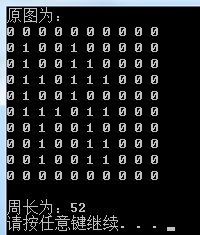展开全文• 周长公式是什么字母公式: C = πD = 2πR公式描述：π是圆周率,约等于3.14,公式中r为圆的半径，d为圆的直径。圆的周长 = 直径× 圆周率 = 半径×2 ×圆周率圆的周长怎么算如圆的直径是50它的周长是多少，是怎么算...
圆周长是指在圆中内接一个正n边形，边长设为an，正边形的周长为n×an，当n不断增大的时候，正边形的周长不断接近圆的周长C的数学现象，即：n趋近于无穷，C=n×an。圆周长公式是什么字母公式: C = πD = 2πR公式描述：π是圆周率,约等于3.14,公式中r为圆的半径，d为圆的直径。圆的周长 = 直径× 圆周率 = 半径×2 ×圆周率圆的周长怎么算如圆的直径是50它的周长是多少，是怎么算的？圆的周长公式：圆的周长C = π X 直径 = π X 半径 X 2 (π=3.14)当圆的直径为50时S=3.14X 50= 157人们在经验中发现圆的周长与直径有着一个常数的比，并把这个常数叫做圆周率。于是自然地，圆周长就是：C = π X 直径或者π X 半径 X 2 。后来的数学家们就想办法算出这个π的具体值，数学家刘徽用的是“割圆术”的方法，也就是用圆的内接正多边形和外切正多边形的周长逼近圆周长，求得圆接近192边型，求得圆周率大约是3.14。什么是圆周率割圆术的大致方法在中学的数学教材上就有。然而必须看到，它很大程度上只是计算圆周率的方法，而圆周长是C = π * d似乎已经是事实了，这一方法仅仅是定出π的值来。仔细想想就知道这样做有问题，因为他们并没有从逻辑上证明圆的周长确实正比于直径，更进一步说他们甚至对周长的概念也仅是直观上的、非理性的。
展开全文• Surround the Trees Time Limit: 2000/1000 MS (Java/Others)Memory Limit: 65536/32768 K (Java/Others) Total Submission(s): 14377Accepted Submission(s): 5559 Problem ...
                          Surround the Trees

Time Limit: 2000/1000 MS (Java/Others)    Memory Limit: 65536/32768 K (Java/Others)
Total Submission(s): 14377    Accepted Submission(s): 5559

Problem Description

There are a lot of trees in an area. A peasant wants to buy a rope to surround all these trees. So at first he must know the minimal required length of the rope. However, he does not know how to calculate it. Can you help him?
The diameter and length of the trees are omitted, which means a tree can be seen as a point. The thickness of the rope is also omitted which means a rope can be seen as a line.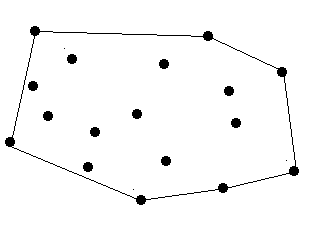There are no more than 100 trees.

Input

The input contains one or more data sets. At first line of each input data set is number of trees in this data set, it is followed by series of coordinates of the trees. Each coordinate is a positive integer pair, and each integer is less than 32767. Each pair is separated by blank.
Zero at line for number of trees terminates the input for your program.

Output

The minimal length of the rope. The precision should be 10^-2.

Sample Input

 

9 12 7 24 9 30 5 41 9 80 7 50 87 22 9 45 1 50 7 0

Sample Output

 

243.06

#include <stdio.h>
#include <math.h>
#include <algorithm>
#include <iostream>
using namespace std;
struct point
{
int x,y;
} p,res,q;
int cross(point a, point b, point c)
{
return (b.x-a.x)*(c.y-a.y)-(c.x-a.x)*(b.y-a.y);
}
int dis(point a)
{
return (a.x-p.x)*(a.x-p.x)+(a.y-p.y)*(a.y-p.y);
}
double dis2(point a,point b)
{
return (a.x-b.x)*(a.x-b.x)+(a.y-b.y)*(a.y-b.y);
}
int cmp(point a, point b)//极角排序
{
if(cross(p, a, b) > 0||(cross(p, a, b)==0&&dis(a) < dis(b)))
return 1;
return 0;
}
double Graham(point *p,point *res, int n)
{
int t = 0;
for(int i = 1; i < n; i++)
{
if(p[t].y > p[i].y || (p[t].y == p[i].y) && p[t].x > p[i].x)
t = i;
}
point temp = p[t];
p[t] = p;
p = temp;
sort(p + 1,p + n,cmp);
res = p;
int top = 0;
for(int i = 1; i < n; i++)
{
while(top > 0 && cross(res[top-1], p[i],res[top]) >= 0)
top--;
res[++top] = p[i];
}
}
double perimeter(int top)
{
double ans=0.0;
for(int i=0; i<top; i++)
{
ans+=sqrt(dis2(res[i],res[(i+1)%top]));
}
return ans;
}
int main()
{
int t;
while(~scanf("%d",&t)&&t!=0)
{
q.x=1010,q.y=1010;
for(int i=0; i<t; i++)
cin>>p[i].x>>p[i].y;
if(t==1)
{
printf("0.00\n");
continue;
}
else if(t==2)
{
printf("%.2lf\n",dis2(p,p));
continue;
}
sort(p,p+t,cmp);
int top=Graham(p,res,t);
double ans=0.0;
ans=perimeter(top);
printf("%.2f\n",ans);
}
return 0;
}


展开全文• 圆的周长公式：c=2πr=πd。公式中r为圆的半径，d为圆的直径。人们在经验中发现圆的周长与直径有着一个常数的比，并把这个常数叫做圆周率π。怎么算圆的周长圆的周长=圆周率×直径c=πd圆的周长=圆周率×2×半径c=2...
圆的周长公式：c=2πr=πd。公式中r为圆的半径，d为圆的直径。人们在经验中发现圆的周长与直径有着一个常数的比，并把这个常数叫做圆周率π。怎么算圆的周长圆的周长=圆周率×直径c=πd圆的周长=圆周率×2×半径c=2πr1.到定点的距离等于定长的点的集合叫做圆。这个定点叫做圆的圆心，通常用字母“o”表示。2.连接圆心和圆周上任意一点之间的连线叫做半径，通常用字母“r”表示。3.通过圆心并且两个端点都在圆周上的线段叫做直径，通常用字母“d”表示。什么是圆周率数学家们想办法算出这个π的具体值，数学家刘徽用的是“割圆术”的方法，也就是用圆的内接正多边形和外切正多边形的周长逼近圆周长，求得圆接近192边型，求得圆周率大约是3.14。割圆术的大致方法在中学的数学教材上就有。然而必须看到，它很大程度上只是计算圆周率的方法，而圆周长是C =π*d似乎已经是事实了，这一方法仅仅是定出π的值来。仔细想想就知道这样做有问题，因为他们并没有从逻辑上证明圆的周长确实正比于直径，更进一步说他们甚至对周长的概念也仅是直观上的、非理性的。
展开全文• 同基里的圆面积（或周长）是椭圆面积（或周长）的面积（或周长）的界。 ③抛物面：长轴相等的抛物面是同基抛物面。两轴 相等的抛物面面积为同基抛物面的界。两轴相等抛 物面的弧长，为同基抛物面弧长界。 ④椭圆球：...
• 梯形是只有一组对边平行的四边形，平行的两边...@视觉中国梯形的周长公式是L=a+b+c+d，其中L代表梯形的周长，变量a是梯形上底边的边长，变量b是梯形下底边的边长(在梯形中，平行的两条边是梯形的底边，短的一条是...
• 梯形的周长怎么计算1、已知两条侧边长和上、下底边长(1)写出梯形的周长公式周长公式是L=a+b+c+d，其中L代表梯形的周长，变量a是梯形上底边的边长，变量b是梯形下底边的边长(在梯形中，平行的两条边是梯形的底边，短...
• 多边形内角计算公式与半径无关 要已知正多边形边数为N 内角和=180(N-2) 半径为R 圆的内接三角形面积公式:(3倍根号3)除以4再乘以R方 外切三角形面积公式:3倍根号3 R方 外切正方形:4R方 内接...计算几何 hdu
• 一个返回多边形周长的方法getPerimeter()。 一个返回多边形面积的方法getArea()。计算正多边形面积的公式是：面积=(n*side2)/(4*tan(180/n)) 分别使用无参构造方法、 RegularPolygon (6,4)和RegularPolygon(10,4,...
• 周长，即用（x的平方加y的平方）开根号即可获得，累计相加，应注意的是，不要忘记最后一个点与起始点的线段。 附代码：顶点数据x y 输入到1.txt文件中，如10 40 #include <vector> //#include <opencv2\...c++
• 三角形、长方形、正方形、梯形、圆等的周长计算公式和面积计算公式? 周长：Girth 面积：Area 1、三角形(一般三角形，海伦公式) Triangle 周长L = a + b + c(a,b,c为三角形的三个边的长，下同) 面积S ...
• 前几天发布了一个关于多边形面积周长计算的博客，由于老师不按常理出牌，因为交作业时没人做，老师又加了梯形和平行四边形，本想着不改了直接交上去得了，可博主偏偏是一个追求完美的人，再加上先前编写的那个程序...java
• 这是一个可以在平面坐标系中表示任意多边形并且计算其面积和周长的类。不过目前只能在第一象限计算。使用时较简便，只要把多边形的各个顶点传进去就可以了（不用按顺序）。 （其中面积的计算是参考...
• //计算多边形周长 double perimeter() { double sum=0; int n=a.size(); for(int i=0;i;i++) sum+=dist(a[i],a[(i+1)%n]); return sum; } //计算多边形的面积 double getDArea() { int n = a.size();...
• 圆内接正多边形算pi的值 先从内接正方形开始，然后扩展到八边形，16边形。。。 通过找出规律，从而计算 4边形时 pi/2=2*1* { 1/(sqrt 2) } 在这里cos s=1/(sqrt 2) ，为45度 8...扩展
• 多边形面积 多边形通常分为凸多边形和凹...最常见的多边形面积计算公式公式表达式为： S= sqrt( p*(p-a)*(p-b)*(p-c)) 其中p为此三角形的半周长，而a,b,c为三角形三边长 若三角形三点为x1,y...
• * 计算多边形面积公式 * * @author Lion Li * @date 2020-05-07 */ public class PolygonArea { public static void main(String[] args) { //多边形面积 double sum = 0; //临时变量 ...java 算法
• OpenCV轮廓、多边形逼近、关键点、周长和面积、边界框、矩、轮廓树、凹凸包、几何直方图、匹配 http://lib.csdn.net/article/opencv/31081 1.轮廓的多边形逼近  2.轮廓的关键点  3.轮廓的周长和...
• public class polygon extends JFrame{ //继承JFrame类 public polygon () { setTitle("多边形的面积与周长计算"); setLayout(null); //绝对布局 setBounds(400,200,660,480); //设置窗体出现位置以及窗体...swing java
• 计算公式公式:http://en.wikipedia.org/wiki/Centroid#Centroid_of_polygon 多边形的质心： 一个非自相交的n个顶点的多边形(x0,y0...在这些公式中，顶点被假定为沿多边形周长编号。此外，顶点（xn，yn）与（x0，y0...
• 多边形的面积可通过分割成很多个三角形面积之和来求得！ 通过多边形各顶点坐标可以求得各边长，再采用海伦公式，计算分割后的小三角形的面积。 ...海伦公式如下： ...公式中的p为半周长： p=(a+b+c)/2c
• 问题描述： 很久以前推送过这样一篇文章， Python使用matplotlib绘制正多边形逼近圆周 ...如果使用正多边形模拟圆周的话，把正多边形周长和外接圆的半径带入上面的公式，可以得到圆周率的近似值，边数越...python 可视化
• 对输入的n个顶点来说，只用得到这n个顶点中的三个顶点，使其构成的三角形周长之和最小，其他的就啥也不用管，进行下一次循环就行了。 这个是网上找到的递推公式。 首先，从n个顶点中找到，三个顶点。 用一个嵌套的...算法 动态规划 java
• 多边形面积 【问题描述】 给出平面上一组顶点的坐标，计算出它们所围成的凸多边形的面积. 输入数据表示了如图所示的四边形。其面积为5.00。 评分标准： 本程序允许使用数学库函数，如果你的输出与标准...
• 题目 Input 输入数据包含多个测试实例，每个测试实例占一行，每行的开始是一个整数n(3<=n<=100)，它表示多边形的边数（当然也是顶点数），然后是按照逆时针顺序给出的n个顶点的坐标（x1, ...多边形面积公式：S=0算法
• 假设在平面内，有一个三角形，边长分别为a、b、c，三角形的面积S可由以下公式求得：S=√[p(p-a)(p-b)(p-c)]而公式里的p为半周长：p=(a+b+c)/2注：'Metrica'(《度量论》)手抄本中用s作为半周长，所以S=√[p(p-a)(p-b)...
• 计算多边形面积 三角形的面积 1、在解析几何里， △ABC的... 海伦公式: S=sqrt[p(p-a)(p-b)(p-c)],其中p=(a+b+c)/2(p为三角形的半周长) 2、 计算几何的方法  △ABC的面积就是“向量AB”和“向量AC”两个向...